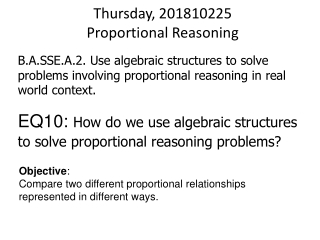DownloadDownload PresentationThursday, 201810225 Proportional Reasoning

# Thursday, 201810225 Proportional Reasoning

Télécharger la présentation## Thursday, 201810225 Proportional Reasoning

- - - - - - - - - - - - - - - - - - - - - - - - - - - E N D - - - - - - - - - - - - - - - - - - - - - - - - - - -
##### Presentation Transcript

1. B.A.SSE.A.2. Use algebraic structures to solve problems involving proportional reasoning in real world context. Thursday, 201810225Proportional Reasoning EQ10: How do we use algebraic structures to solve proportional reasoning problems? Objective: Compare two different proportional relationships represented in different ways.

2. Thursday, 20181025 Essential Questions • EQ10: How do we use algebraic structures to solve proportional reasoning problems? Vocabulary: • Unit rates • Fractions • Solving Proportions • Ratios • Finding percent and calculating percent of a number • Determining constant of proportionality (slope) from tables of values, graphs, and algebraic relations • Interpreting constants of proportionality (slope) on a graph • Proportional Reasoning Agenda Bridge Math • Store your phones • Find your seat • Bellwork: Percents • Calculator • Proportional reasoning problems/ Group Stations • Exit Ticket: Factor Puzzle • Remain in seat until bell rings. Teacher Dismisses NOT the bell. No Sleeping! It’s always a great day to be a Wolverine!

3. Bellwork: Thursday. 20181025Percents – Start Unknown • _____ is 5% of 40 • _____ is 100% of 40 • _____ is 200% of 40 • _____ is 1% of 40 • _____ is 50% of 40 • _____ is 6% of 40 • _____ is 25% of 40 • _____ is 0.5% of 40 • _____ is 10% of 40 • _____ is 13.5% of 40

4. Remember…. • A ratio is a comparison of two quantities by division. • A ratio compares part to part or part to whole. • A ratio can be written 3 different ways… n/d n:d or “n to d” or “n out of d”

5. B.A.SSE.A.2. Use algebraic structures to solve problems involving proportional reasoning in real world context. Monday, 20181022Proportional Reasoning EQ10: How do we use algebraic structures to solve proportional reasoning problems?

6. EQ9 Proportional Reasoning/Ratios That's All Folks! Get Ready for the EXIT TICKET!!!

7. Factor Puzzles EXIT TICKET

8. Answer: Factor Puzzles 3 7 2 15 5

9. Tidy Up- LEAVE WORK OUT • Selected people will • carry out the following • tasks: • Collect felts and put back in box • Collect pencils and put back • Collect help sheets/ templates and put in middle of your table • EVERYONE ELSE STAYS IN THEIR SEAT!!!!!!!